# Ultimate parallax tutorial (with tool) (Read 1253 times)

Started by Master Yoda, March 09, 2019, 10:58:22 pm
Share this topic:

#### Master Yoda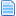##### Ultimate parallax tutorial (with tool)
#1  March 09, 2019, 10:58:22 pm
••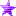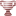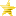This tutorial is an extension of the original made by @VIB: http://mugenguild.com/forum/topics/parallax-tutorial-vib-116138.msg1119796.html#msg1119796
Thanks to @Odb718 for helping me with the translation: http://mugenguild.com/forum/topics/parallax-tutorial-translation-english-184639.msg2416662.html#msg2416662

The idea is to introduce you to the basic concepts, but you can calculate them automatically with this tool: http://mugenguild.com/forum/topics/parallax-calculator-tool-186212.new.html#new

1. PARALLAX BASICS
Parallaxing background elements give the illusion of depth to elements that appear to move in front of each other due to depth.
There are two different parameters to achieve this effect, both are mutually exclusive:

1.1. XSCALE
Code:
`XSCALE = top_xscale, bottom_xscale`
Our parameter xscale, is composed of: top_xscale and bottom_xscale.
The first parameter scales the horizontal delta of the background element's top edge.
The second xscale parameter scales the horizontal delta of the background element's bottom edge.
The deltas for the rest of the element are linearly interpolated between these two values. (The computer figures out the rest)
Code:
`[BG my_parallax_element]type = parallaxsprite = 3, 0delta = .75, .9xscale = 1, 2mask = 1`
For example, delta = .75, .1 and xscale = 1, 2 specifies the top of the sprite would move at .75 * 1 = .75 stage units per camera unit, and the bottom would move at .75 * 2 = 1.5 stage units per camera unit.

The "top_xscale" factor will always be equal to 1 for the purpose of simplifying the calculations, so all times we must determine the value of "bottom_xscale".

Top_xscale and bottom_xscale respectively scale the horizontal delta of the background element's top and bottom edge to create a horizontal shearing effect.

Use xscale if the sprite has already been made for perspective distortion.
This effect is the one used by most cps2 games that have a parallax effect: SF, Darkstalkers, etc.

> This is how the sprite looks in the sff> and so it would look in game> the arrows indicate the direction in which the image is contracted1.2 WIDTH
Code:
`WIDTH = top_width, bottom_width`
Top_width and bottom_width respectively specify the top and bottom widths of a trapezoid that has the same height as the sprite used. The sprite will be distorted to match the shape and size of the trapezoid. The ratio of top_width to bottom_width affects the amount of shearing as the camera moves horizontally.
Use width if the sprite is not made for perspective distortion. For example:

> This is how the sprite looks in the sff> and so it would look in game> the arrows indicate the direction in which the image expands2. GENERAL PARAMETERS
For the development of this tutorial, we will use the SF II Honda stage as a base. It has all the elements we need. We will start by identifying the basic parameters, and first developing the formula to apply it to the XSCALE factor, and then transfer it to the WIDTH factor,WHERE:
• h is the size of the sprite (in pixels)
• A is the distance between the top and the char line
• B is the distance between char line and bottom
• zoffset = is the vertical distance of the ground level from the top of the screen, given in pixels.
Another important point is the dimension of the stage. Which will obviously depend on the sprites. As of version 1.0, when supporting higher resolutions (the winmugen only supported 320x240), a new parameter was introduced, the localcoord. It's used to "tell" the engine, which is the pixel resolution of the stage that is showing on screen.
According to the mugen docs:
• localcoord = width, height. Dimensions of the coordinate space of the stage. Defaults to 320, 240 if omitted.
We are interested in the second value of the localcoord parameter: "height", since it is the one that directly affects the zoffset. If our "height" in pixels is 240 (localcoord = 320,240) our zoffset, which will be represented by the char line, could in principle assume any value within that range.

3. PROGRAMING OUR CODES
Having defined the basic concepts, we will begin to work. For this we will start with the formula developed by VID:
bottom_xscale = ((1-xdelta) * B / A + 1) / xdelta

3.1 DETERMINE THE LOCALCOORD
First, we must determine the resolution of the sprites. In our example, because it is an LR stage, this will be: 320 x 240. The number 240, will affect the zoffset.
Code:
`Localcoord = 320,240`
3.2 DEFINE THE ZOFFSET
Next, we must record the value of the zoffset, which will depend on:
• the source material in case it is a port,
• taste of the programmer, or
• of the desired effect.
I using the source material, in that case the SF II game.
[/list]
Code:
`Zoffset = 216 `

3.3 DEFINE THE XDELTA
The same criterion applied to the zoffset, will be used for the value of the xdelta. Again, using the original source: Xdelta = 0. 67
Code:
`delta= .67, 0`

3.4 DETERMINE THE HEIGHT OF THE SPRITE FOR THE FLOOR: "h" factor
Subsequently, you must determine the height of the floor.
In the Honda stage, the height is 66 pixels.It remains to determine the last values:
h = A + B

Where:

B= Heigth – zoffset
B = 240 -216
B = 24

A = h – B
A = 66 – 24
A = 42

3.5 CALCULATE XSCALE
Code:
`XSCALE = top_xscale, bottom_xscale`

Replace the values in the formulabottom_xscale = ((1-xdelta)*B/A+1)/xdelta
bottom_xscale = (1-0.67)*24/42+1)/0.67
bottom_xscale = 1.773987207Our code will then be like this:
Code:
`[BG FLOOR]type  = parallaxspriteno = 3, 0delta = .67, 1xscale = 1, 1.77398720682303mask = 1`
Checking the result3.6 CALCULATE WIDTH
Code:
`WIDTH = top_width, bottom_width`

For this case, we will follow all the points described above (3.1 to 3.5), only that we will need to calculate the bottom_width.

"top_width", it's similar to "top_xscale". The first is expressed in delta and the second in pixels of width of the sprite
"bottom_width", it's similar to "bottom_xscale". The first is expressed in delta and the second in pixels of width of the sprite

The "top_width" factor will always be equal to width in pixels of the floor sprite for the purpose of simplifying the calculations, so all times we must determine the value of "bottom_width".

Then:
top_width= 696 (width in pixels)
bottom_width= top_width * bottom_xscale
bottom_width= 696*1.77398720682303
bottom_width= 1234.6951 (pixels)
Code:
`[BG FLOOR]type  = parallaxspriteno = 3, 0delta = .67, 1width = 696, 1234.6951mask = 1`

3.7 POSITIONING AN OBJECT ON THE FLOOR WITH PARALLAX
In this case, you must determine the h1 factor.WHERE:
• h1 is the distance between the top of the floor sprite and the base of the object's sprite (in pixels)

Remembering that
X delta top= 0,67
X delta bottom= 1,1885
h = 66 pixels

Delta increased = 1,1885 - 0,67 =0,5185

Variation of the delta for each pixel unit= 0,5185/66= 0,0078

Delta objet= (Variation of the delta for each pixel unit * h1)+X delta top
= ( 0,0078 * 19) + 0,67
= 0,8193
Code:
`[BG BATHTUB_FRONT]type= normalspriteno= 4,0delta = 0.819285714285714, 1mask= 1`

3. USING THE TOOL
Determine your values of localcord, zoffset, xdelta and h. Add them in the excel file and magic.
Last Edit: March 10, 2019, 03:05:18 am by Master Yoda

#### O Ilusionista##### Re: Ultimate parallax tutorial (with tool)
#2  March 09, 2019, 11:21:23 pm

#### Master Yoda##### Re: Ultimate parallax tutorial (with tool)
#3  March 09, 2019, 11:29:14 pm
••#### 2Dee4ever##### Re: Ultimate parallax tutorial (with tool)
#4  March 09, 2019, 11:50:30 pm
•••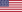I am going to study this further. Rather than just guessing for a lot of values. Thank you for taking the time to type it all out.
Spoiler, click to toggle visibilty

#### Master Yoda##### Re: Ultimate parallax tutorial (with tool)
#5  March 10, 2019, 03:06:57 am
••I am going to study this further. Rather than just guessing for a lot of values. Thank you for taking the time to type it all out.
Thanks man!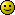#### Stone-Wolf##### Re: Ultimate parallax tutorial (with tool)
#6  March 10, 2019, 03:53:58 am
••• i'm not rude, google translate is
•whoa, is just what i was looking for, thanks man#### beterhans##### Re: Ultimate parallax tutorial (with tool)
#7  March 10, 2019, 06:50:03 am
••I tested yesterday

For example, delta = .75, .1 and xscale = 1, 2 specifies the top of the sprite would move at .75 * 1 = .75 stage units per camera unit, and the bottom would move at .75 * 2 = 1.5 stage units per camera unit.

is not true

delta = .75, .1
0.75 is upline delta

xscale = 0.5, 1
xscale = 1, 2
xscale = 2, 4
have no difference.

Tested in mugen 1.1

My MUGEN Website ==> for All my Characters Stages and Articles
https://beterwork-us.hopto.org

#### Master Yoda##### Re: Ultimate parallax tutorial (with tool)
#8  March 10, 2019, 03:51:34 pm
••whoa, is just what i was looking for, thanks man

Your welcome! I hope you find it useful!

I tested yesterday

For example, delta = .75, .1 and xscale = 1, 2 specifies the top of the sprite would move at .75 * 1 = .75 stage units per camera unit, and the bottom would move at .75 * 2 = 1.5 stage units per camera unit.

is not true

delta = .75, .1
0.75 is upline delta

xscale = 0.5, 1
xscale = 1, 2
xscale = 2, 4
have no difference.

Tested in mugen 1.1

I see you have misinterpreted a couple of points:
The general idea is that in the line of zoffset your xdelta = 1 to avoid the displacement of the chars.
If you double or triple the values of the xcale will affect the xdelta of the zoffset. Let me exemplify it with the codes of the honda stage.

Normal code:
Code:
`[BG FLOOR]type  = parallaxspriteno = 3, 0delta = 0.67, 1xscale = 1, 1.77398720682303mask = 1`

So:And your xdeltas:
xdelta top= 0,67* 1= 0,67
xdelta bottom= 0,67*1,77=1,18
xdelta zoffset= 1Now let's duplicate the xscale values as in your example= *2

Code:
`[BG FLOOR]type  = parallaxspriteno = 3, 0delta = 0.67, 1xscale = 2, 3.547974414mask = 1`

SoBut now your xdeltas:
xdelta top= 0,67* 2= 1,34
xdelta bottom= 0,67*3,54=2,37
As you can now check xdelta in your zoffset is equal to 2. Then the characters will slide across the floorYou can not change the values randomly in the formula and expect it to work.
The formula was specifically designed keeping in mind that the xdelta of its zoffset should be equal to 1 to avoid displacements of the characters.

Also always remember this:
The "top_xscale" factor will always be equal to 1 for the purpose of simplifying the calculations, so all times we must determine the value of "bottom_xscale".

Greetings!
Last Edit: March 10, 2019, 09:15:20 pm by Master Yoda

#### beterhans##### Re: Ultimate parallax tutorial (with tool)
#9  March 11, 2019, 12:41:12 am
••My MUGEN Website ==> for All my Characters Stages and Articles
https://beterwork-us.hopto.org
Last Edit: March 11, 2019, 12:44:42 am by beterhans

#### Master Yoda##### Re: Ultimate parallax tutorial (with tool)
#10  March 11, 2019, 02:05:28 am
••I understand your point but for me
No matter I change the value the stage will be ok no sliding will happen.

I wonder which version of mugen you are using?
1.0?

I have tested in version 1.1 and you are right. The strange thing is that the MUGEN DOCS historically said the same thing:
xscale = top_xscale, bottom_xscale ; (float, float) top_xscale and bottom_xscale respectively scale the horizontal delta of the background element's top and bottom edge to create a horizontal shearing effect. For example, delta = .75, .75 amd xscale = 1, 2 specifies the top of the sprite would move at .75 * 1 = .75 stage units per camera unit, and the bottom would move at .75 * 2 = 1.5 stage units per camera unit. This example assumes the sprite axis is at the top of the sprite.
Maybe it's an error in the update of the engine.
In this case, the xdelta will not be affected by the top_x scale,

Anyway, always your top_x scale  will be equal to 1 (top_x scale=1), and you will have to continue calculating the bottom_x_scale in the same way.
Last Edit: March 11, 2019, 02:24:29 am by Master Yoda

#### beterhans##### Re: Ultimate parallax tutorial (with tool)
#11  March 11, 2019, 03:59:06 am
••Anyway, always your top_x scale  will be equal to 1 (top_x scale=1), and you will have to continue calculating the bottom_x_scale in the same way.

by this way
you don't need to make 1st value of xcale to be 1
you can really input the upper delta into the 1st one and the botton delta into the 2nd one and it works
no calculating is needed.

you can view my video next to this post. it will be much easier.
My MUGEN Website ==> for All my Characters Stages and Articles
https://beterwork-us.hopto.org

#### Master Yoda##### Re: Ultimate parallax tutorial (with tool)
#12  March 13, 2019, 12:58:07 pm
••Anyway, always your top_x scale  will be equal to 1 (top_x scale=1), and you will have to continue calculating the bottom_x_scale in the same way.

by this way
you don't need to make 1st value of xcale to be 1
you can really input the upper delta into the 1st one and the botton delta into the 2nd one and it works
no calculating is needed.

you can view my video next to this post. it will be much easier.
I'm going to take a look at your video.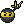#### MatreroG##### Re: Ultimate parallax tutorial (with tool)
#13  March 14, 2019, 03:20:35 am
All this is very useful and I think it will solve some of my problems.
Thanks.